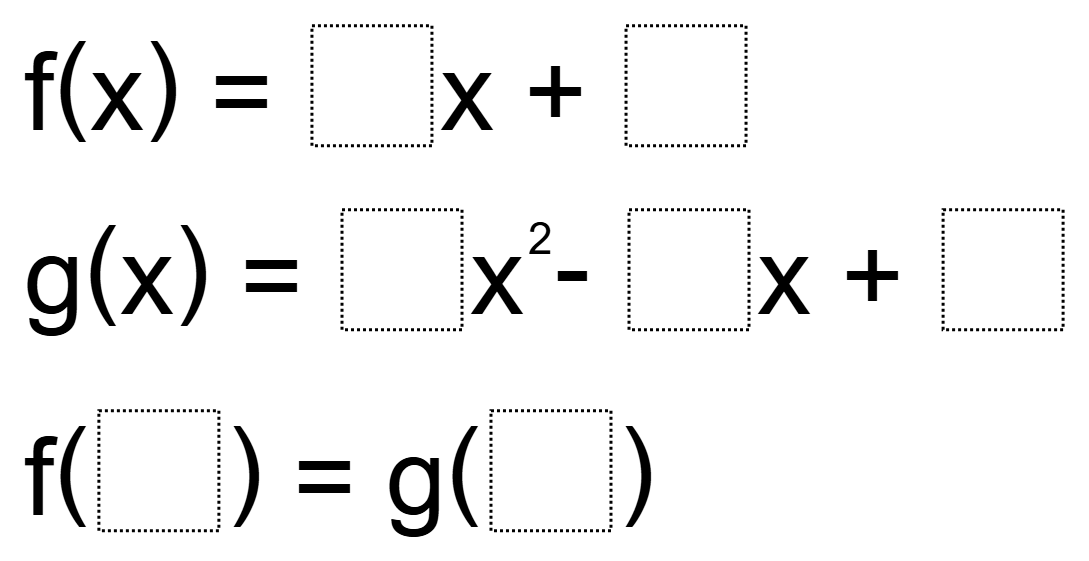Home > High School: Functions > Function Notation

# Function Notation

Directions: Using the digits 1 to 9, at most one time each, fill in the boxes so that the two functions are equivalent### Hint

How do you use function notation to determine the input and output of a function?

f(x) = 9x + 4
g(x) = 7x^2 – 1x + 5
f(3) = g(2)

Post any others in the comments.

Source: Steven Midzak

## Creating Sequences

Directions: Using the digits 1-9, at most one time each, complete the first three terms …

1.I think the format is confusing, and the answer uses incorrect notation and the two functions are, in fact, never “equivalent”. Rephrase like this?

Fill in spaces with whole numbers 1 – 9 only one time, so that the final equality is correct.
f(x) = ___ x + _____
g(x) = ___ x^2 – ____x + _____
f(____) = g(_____)

•I agree. I like the format you used better Tim.

•Thank you for this suggestion. I’ve edited the question accordingly.

•It doesn’t appear to be edited.

2.f(3) = 2x + 4
g(1) = 7x^2 – 6x + 9

3.F(3)=9x+4 g(2)=7x^2-1x+5

4.5.Katy Howell Escobar

𝑓(3)=7𝑥+6 𝑔(2)=5𝑥^2−1𝑥+9

𝑓(8)=6𝑥+4 𝑔(5)=2𝑥^2−1𝑥+7

6.I gave this to my IM3 classes today, here are some they came up with that are NOT listed above:

f(3) = 6x + 1 g(4) = 2x^2 – 5x + 7
f(8) = 2x + 3 g(6) = 1x^2 – 4x + 7
f(1) = 3x + 6 g(2) = 4x^2 – 8x + 9
f(2) = 3x + 4 g(1) = 7x^2 – 5x + 8
f(2) = 3x + 7 g(5) = 1x^2 – 4x + 8

7.I should have looked at the solutions earlier. That negative before the x term in the quadratic makes all the difference as per Tim’s comment waaaaay back. I wonder why the question as posted still shows the +.

Thank you for this site, it is awesome!

8.f(x) = 8x + 5; g(x) = 3x^2-x+9
f(6) = g(4) = 53